#### You may also like### Cubes

How many faces can you see when you arrange these three cubes in different ways?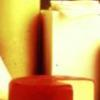### The Big Cheese

Investigate the area of 'slices' cut off this cube of cheese. What would happen if you had different-sized block of cheese to start with?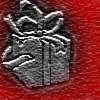### Wrapping Presents

Choose a box and work out the smallest rectangle of paper needed to wrap it so that it is completely covered.

##### Age 7 to 11Challenge Level

Well, you have a whole collection of cubes and we're going to paint the outside that's visible once we've put them together.
A few simple rules:
• the cubes must be together;
• they must not be toppling over.
We'll need to look at how much paint is needed. One Brush Load (a kind of unit that we'll use) will paint one square face.
Well here are $5$ cubes: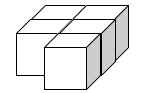Counting the faces to be painted comes to $15$, so $15$ Brush Loads (remember we're only counting visible faces, so not those that are touching the surface where the cubes are placed).

But of course we could have placed the $5$ cubes differently: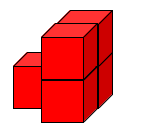Counting the faces to be painted we now have $17$, so $17$ Brush Loads.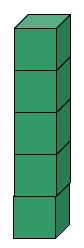Now we'll need $21$ Brush Loads.

O.K. Now let's explore different numbers of cubes, say $6, 7, 8, 9,$ etc. Each time we count the number of square faces showing and so find the number of Brush Loads (BLs).
The cubes - as in the case of $5$ - can be arranged in different ways, sometimes giving different BLs.

So I'm setting the challenge of finding ways of arranging cubes so that first of all there are the least number of BLs needed and then the biggest number of BLs needed.

Try first with $5$ cubes.
How about $6, 7, 8$ and $9$ cubes?

If you wish to go further, then increase the number of cubes. See if you can get all the numbers between the largest and the smallest e.g. if $15$ is the smallest number for $5$ cubes and $21$ is the largest, can you get arrangements that'll give $16, 17, 18, 19,$ and $20$ BLs?

### Why do this problem?

This activity is very practical, and encourages visualisation. It can help pupils' development of the concepts associated with area - surface area. It is an investigation that can be extended very far indeed, and some pupils may succeed beyond your expectation.

### Possible approach

It is worthwhile for pupils to work in pairs or threes and to have cubes in front of them so as to enable good discussions to take place. The youngsters usually find that keeping shapes flat produces smaller numbers and making the tallest towers produces the largest, but challenge them to explain why this is the case. Look out for children moving one cube about from one place to another - you can get into good quality conversations about how many square faces are being covered by the new position and how many new square faces are added.

Some younger children find it hard to count the faces that are visible, and they recount some or miss some out - don't we all? The children have to invent ways of being more and more accurate. It's good to ask them about their ways of counting and you may very well get these kinds of replies: "I look at each cube in turn and count the faces that are visible" or "Well I go all around the edge then count the ones on top". So for example with these $8$ cubes: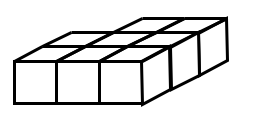they'd count "$3$ plus $3$ plus $3$ plus $3$ plus $8$". It then becomes very interesting that with $9$ cubes there will also be $4$ threes but adding $9$ at the end. Moving to $10$ cubes it would be $3 + 4 + 3 + 4 + 10$. The discussions can go on and on as the confidence grows.

### Key questions

How are you counting the faces?
Are you able to make a shape with more/fewer faces?
Can you explain why or why not?

### Possible extension

The more mature youngsters who have no difficulties in counting will probably move on to tabulating their results. You might want to use standard units (cm$^2$) with older children. If you use multilink, $1$BL might be equivalent to $4$ cm$^2$. Here is a spreadsheet of some findings related to this investigation that you may find useful. But, please don't just hand it out to the children!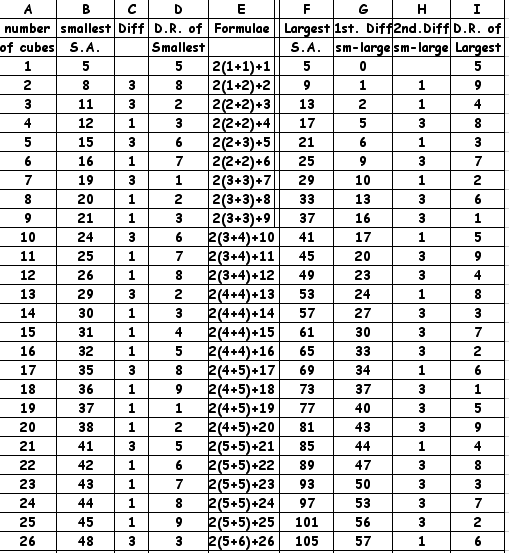You might like to read the article Back to the Practical? which uses this problem as an example.

### Possible support

Probably the best way to support learners who are struggling is to make sure they have a supply of cubes and encourage them to discuss what they are doing.